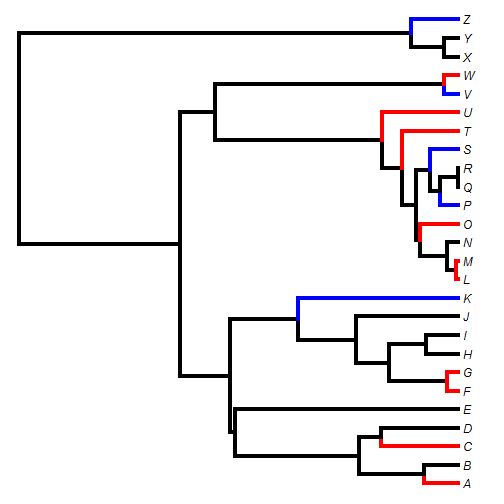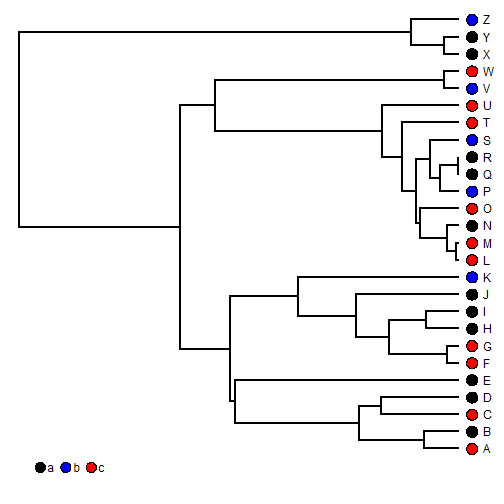## Tuesday, January 10, 2017

### Plotting terminal edges of the tree different colors depending (or not) on a discrete character using phytools

I recently received the following request:

“I would love to know if there is any way of extracting and colouring only a certain set of the terminal edges of the phylogeny, based on the tip labels. For example, using edge.color within plot.phylo based on a vector of tips. Do you know of any code that could be used to do this.”

This is pretty straightforward to do using phytools. Note that it can also be done using `plot.phylo` in the ape package, but I'm going to focus on the phytools way for obvious reasons.

I'll image that I'm painting the terminal edges leading to each tip with different colors based on the state of a discrete trait - but we could modify our technique for any arbitrary grouping of tips.

Here's the tree & data:

``````library(phytools)
tree
``````
``````##
## Phylogenetic tree with 26 tips and 25 internal nodes.
##
## Tip labels:
##  A, B, C, D, E, F, ...
##
## Rooted; includes branch lengths.
``````
``````x
``````
``````## A B C D E F G H I J K L M N O P Q R S T U V W X Y Z
## c a c a a c c a a a b c c a c b a a b c c b c a a b
## Levels: a b c
``````

Now, let's say we want to color the edges blue & red respectively if the corresponding edge is in state `b` or `c`, respectively, and leave it black if the tip is in state `a`.

``````## identify the tips in states b or c
b<-names(x)[x=="b"]
b
``````
``````##  "K" "P" "S" "V" "Z"
``````
``````c<-names(x)[x=="c"]
c
``````
``````##   "A" "C" "F" "G" "L" "M" "O" "T" "U" "W"
``````
``````## paint the edges
tt<-paintBranches(tree,edge=sapply(b,match,tree\$tip.label),
state="b",anc.state="a")
tt<-paintBranches(tt,edge=sapply(c,match,tree\$tip.label),
state="c")
``````

Now we can plot our tree:

``````tt
``````
``````##
## Phylogenetic tree with 26 tips and 25 internal nodes.
##
## Tip labels:
##  A, B, C, D, E, F, ...
##
## The tree includes a mapped, 3-state discrete character with states:
##  a, b, c
##
## Rooted; includes branch lengths.
``````
``````cols<-setNames(c("black","blue","red"),c("a","b","c"))
plot(tt,colors=cols,lwd=4,split.vertical=TRUE,ftype="i")
``````Let's check this against a plot generated using `dotTree`:

``````dotTree(tree,x,colors=cols)
``````Tree & data for this demo were simulated as follows:

``````tree<-pbtree(n=26,tip.label=LETTERS)
Q<-matrix(c(-2,1,1,1,-2,1,1,1,-2),3,3)
rownames(Q)<-colnames(Q)<-letters[1:3]
x<-as.factor(sim.history(tree,Q)\$states)
``````

1.How is the object x created ? How would this work use a tab-delimited file where column 1 is the tip label names and column 2 is the state, where states are defined as 0 and 1 ?

1.You have to make a named character. This is what I did with the first column in plants.csv names and the second column is the trait.
trait <- plants\$V2
names(trait) <- plants\$V1
trait <- as.factor(trait)

2.How did you created x? I have similar data.
Thanks for providing such a nice blog.

3.Hello, Thanks for providing such a nice blog. Your package is magic. Can you please help me to convert this tree into unrooted tree?

Note: due to the very large amount of spam, all comments are now automatically submitted for moderation.